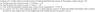# Particle falling radially into a black hole

• Cythermax

#### Cythermax

New poster has been reminded to show their work on schoolwork problems
Homework Statement
Attached it below
Relevant Equations
Not sure which equations necessary here.
I've been stuck starting anywhere with this. I need to finish this class for graduation and i'd like a safety net of a passing grade with this.

#### Attachments

•1121.PNG
9.3 KB · Views: 87
Well, do you know what the Schwarzschild metric is? In Schwarzschild (spherical) coordinates, if a particle starts at the point ##r, \theta, \phi## at time ##t## and travels a small distance to the point ##r+\delta r, \theta + \delta \theta, \phi + \delta \phi## by time ##t+\delta t##, then the the change in proper time ##\delta \tau## satisfies:

##(\delta \tau)^2 = (1 - 2GM/(c^2 r))^{-1} (\delta t)^2 - (1 - 2GM/(c^2 r)) (\delta r)^2 - r^2 \delta \theta^2 - r^2 sin^2(\theta) (\delta \phi)^2##

•